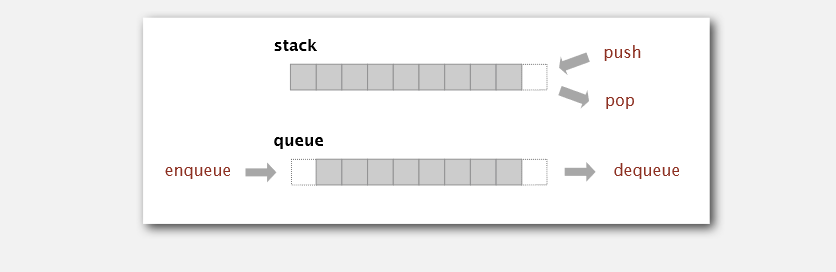# LeetCode-三周攻克数据结构-栈-队列篇2021 年 08 月 08 日

## DAY9

### 1. 有效的括号

``````给定一个只包括 '('，')'，'{'，'}'，'['，']' 的字符串 s ，判断字符串是否有效。

#### 方法1：栈匹配法

1. 建立一个括号的映射: 括号`左边` => 匹配`右边`
2. 遍历判断左边进`栈`
3. 判断右边 取`栈`- 判断是否匹配
``````function isValid(s: string): boolean {
// 奇数直接 返回 false
if (s.length % 2 !== 0) return false

// 新建一个栈
const stack = []
// 映射 三个括号
const map = {
'(':')',
'[':']',
'{':'}',
}

for (let str of s) {
// 是左括号的话 就进栈 判断
if ( str in map ){
stack.push(str)
continue;
}
// 右括号的话，判断栈的最后一个是否匹配【完整的括号】
if (map[stack.pop()] !== str) return false
}

return !stack.length
};``````

### 2. 用栈实现队列

``````你仅使用两个栈实现先入先出队列。队列应当支持一般队列支持的所有操作（push、pop、peek、empty）：

void push(int x) 将元素 x 推到队列的末尾 int pop() 从队列的开头移除并返回元素 int peek() 返回队列开头的元素 boolean empty() 如果队列为空，返回 true ；否则，返回 false

["MyQueue", "push", "push", "peek", "pop", "empty"]
[[], , , [], [], []]

[null, null, null, 1, 1, false]
​

MyQueue myQueue = new MyQueue();
myQueue.push(1); // queue is: 
myQueue.push(2); // queue is: [1, 2] (leftmost is front of the queue)
myQueue.peek(); // return 1
myQueue.pop(); // return 1, queue is 
myQueue.empty(); // return false

1 <= x <= 9 最多调用 100 次 push、pop、peek 和 empty 假设所有操作都是有效的 （例如，一个空的队列不会调用 pop 或者 peek 操作``````

#### 方法1：双栈实现

``````class MyQueue {
// 进栈
public stack: number[] = []
// 出栈
public stackOut: number[] = []

// 吧进栈数据倒入到出栈
inOut (): void {
while (this.stack.length) {
this.stackOut.push(this.stack.pop());
}
}

push(x: number): void {
this.stack.push(x)
}

pop(): number {
if (!this.stackOut.length) {
this.inOut();
}
return this.stackOut.pop();
}

peek(): number {
if (!this.stackOut.length) {
this.inOut();
}
return this.stackOut[this.stackOut.length - 1];
}

empty(): boolean {
return this.stackOut.length === 0 && this.stack.length === 0;
}
}

/**
* Your MyQueue object will be instantiated and called as such:
* var obj = new MyQueue()
* obj.push(x)
* var param_2 = obj.pop()
* var param_3 = obj.peek()
* var param_4 = obj.empty()
*/``````

### 科普篇